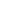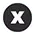## Explore 1+ Data Mining Project Help SolutionsQuestion Number: 564

Project Description The aim of the project is to acclimate you to the process of conducting research in modern data mining or machine learning. You are asked to replicate the existing literature on simple synthetic or small datasets. This is a fundamental ability in your future research or work. Topic DescriptionsTopic 1: Uncertainty-aware Deep LearningIntroduction:MIT 6.S191: Evidential Deep Learning and UncertaintyPractical Uncertainty Estimation & Out-of-Distribution Robustness in Deep Learning Paper:  Simple and Principled Uncertainty Estimation with Deterministic Deep Learning via Distance Awareness Reference code: code Problem 1: What is the problem that the paper aims to solve, and why is this problem important or interesting? Problem 2: What is the meaning of “distance awareness”? How do the authors achieve “distance awareness”? Problem 3: Design and implement a toy experiment to showcase the effect of “spectral normalization”. Problem 4: Reproduce the toy experiment (Figure 1 (f),(i),(j)) in the paper. You need to output the three figures. Problem 5: Conduct the same sets of experiments as Problem 4 on the mixture of 8 Gaussians and 25 Gaussians respectively. These Gaussians can be either identical or with different parameters at your choice. You need to output six pictures.Topic 2: Image Generation with Probabilistic Diffusion Models Introduction:DDPM - Diffusion Models Beat GANs on Image Synthesis (Machine Learning Research Paper Explained)What are Diffusion Models? Paper:Denoising Diffusion Probabilistic Models (DDPM) Reference code: code1 or code2 or code3 Problem 1: What is the problem that the paper aims to solve, and why is this problem important or interesting? Answer this question in two sentences. Problem 2: Implement DDPM and test it on 2-dimensional Swiss roll data. You should output Fig.3 of the blog intro 2. (20 points) Problem 3: Test DDPM on the mixture of 8 Gaussians and 25 Gaussians respectively. These Gaussians can be either identical or with different parameters at your choice. You should output figures like those in Problem 2. Problem 4: Test DDPM on MNIST dataset. Visualize the denoising process (You should output a figure like Fig. 6 in the paper). Problem 5: Perform an interpolation experiment with your trained model from Problem 4 (You should output a figure like Fig. 8 in the paper). Topic 3: Image Classification with Normalizing Flows Introduction:L3 Flow Models -- CS294-158-SP20 Deep Unsupervised Learning -- UC Berkeley -- Spring 2020Flow-based Deep Generative Models Papers: Density estimation using Real NVPSemi-Supervised Learning with Normalizing Flows Reference code: code 1 and code2 Problem 1: Use Real NVP to model the density for the 2-dimensional two-moon, circles, and pinwheel datasets, respectively. You need to output the samples generated from your trained model. You can use code 1 as a library. Problem 2: Use Real NVP to do classification on the two-moon, circles, and pinwheel datasets, respectively. You should output figures similar to those in this notebook demo. Note: the paper  is performing semi-supervised learning, while you are required to do supervised learning where all labels of the data points are available. Problem 3: Use Real NVP to do classification on MNIST dataset. Report the prediction accuracy. Problem 4: Interpolate the latent space to generate pictures between “2” and “9”. Problem 5: Use Real NVP to do classification on CIFAR10 dataset. Report the prediction accuracy. Problem 6: Interpolate the latent space to generate pictures between “cat” and “dog”.

Ans:You can contact with our chat expert in order to get your answer

../

1000+

Students can't be wrong